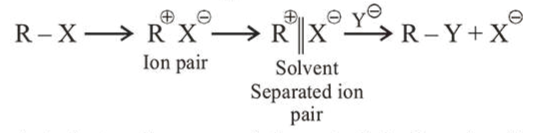# A student writes general characteristics based on the given mechanism as :Question:

The mechanism of $S_{N} 1$ reaction is given as :A student writes general characteristics based on the given mechanism as :

(a) The reaction is favoured by weak nucleophiles.

(b) $R^{\oplus}$ would be easily formed if the substituents are bulky.

(c) The reaction is accompanied by racemization.

(d) The reaction is favoured by non-polar solvents. Which observations are correct?

1. (a) and (b)

2. (a) and (c)

3. (a), (b) and (c)

4. (b) and (d)

Correct Option: , 3

Solution:

Above reaction is $S_{N} 1$ reaction as it proceeds via formation of carbocation. Polar protic solvent is more suitable for $S_{N} 1$ and so racemisation takes place.